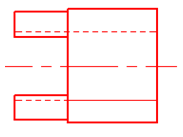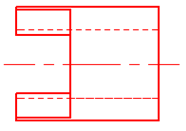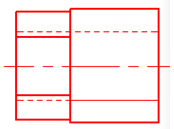HR
【单选题】帝国主义多次通过战争、经济等形式企图瓜分中国，但最后终于还是失败了，根本原因是( )
A.

B.

C.

D.手机使用分享复制链接新浪微博分享QQ微信扫一扫反馈A.
B.

C.

D.

A.

B.

A.

B.

A.

B.

FX系列PLC中有多少个栈存储器（ ）.
A.
8
B.
10
C.
11
D.
16

A.

B.

A.B.C.A.

B.

C.

D.

A.

B.

A.

B.

C.

D.

A.

B.

A.

B.

A.

B.

A.

B.

C.

D.

A.

B.

C.

D.

A.

B.

A.

B.

A.

B.

A.

B.

A.

B.

A.
A
B.
B
C.
C

A.

B.

A.

B.

A.

B.

A.

B.

C.

D.

A.

B.

A.

B.

A.

B.

A.

B.

A.

B.

A.

B.

C.

D.

A.
A
B.
B
C.
C
D.
D

A.

B.

A.

B.
C.

D.

2010年甲公司获准注册了商标“太阳”。2015年甲公司发现乙公司未经许可在同种商品上使用“太阳”商标，于是向法院起诉。2016年法院判决乙公司赔偿甲公司30万元。2017年法院强制执行了15万元。2018年该注册商标被宣告为无效，下列说法正确的有（）
A.

B.

C.

D.

A.

B.

A.

B.

A.

B.

A.

B.

A.

B.

A.

B.

A.

B.

8字形压步是指在压步练习时滑行的路线与8字形相同，这种练习的好处是可以同时练习两个方向，使练习更全面不枯燥并且更流畅。
A.

B.

A.

B.

$设P：2是素数，Q：3是素数，R：\sqrt{2}是有理数，下列命题公式中哪一个的真值为假？$
A.
$(P \vee Q) \rightarrow R$
B.
$R \rightarrow(P \vee Q)$
C.
$(P \wedge Q) \rightarrow P$
D.
$(R \vee Q) \leftrightarrow P$

A.

B.

C.

D.

A.

B.

A.

B.

A.

B.

2016年7月甲公司因侵犯乙公司的商标权，被法院判决赔偿乙公司200万元。甲公司支付150万元的赔偿金后，与乙公司达成协议，2018年10月底前支付剩余50万元，并支付5万元的利息。2018年10月29日甲公司商标被宣告为无效。乙公司要求甲公司返还已支付150万元赔偿金，甲公司则要求乙公司支付尚未支付的50万元及利息。下列说法正确的有（）
A.

B.

C.

D.

$命题公式(p \wedge q) \rightarrow \neg p的成真赋值为?$
A.
$p=0,q=0$
B.
$p=0,q=1$
C.
$p=1,q=0$
D.
$p=1,q=1$
$P \leftrightarrow \neg Q \Leftrightarrow ()$
A.
$\neg P \rightarrow(P \rightarrow Q)$
B.
$\left(\neg P \vee Q\right) \wedge(\neg Q \vee P)$
C.
$(\neg P \vee \neg Q) \wedge(\neg Q \vee P)$
D.
$(\neg P \vee \neg Q) \wedge (Q \vee P)$

A.

B.

A.

B.

A.

B.

A.
ωx=0
B.
ωy=-ωcosγ
C.
ωz=ωsinγ
D.

A.

B.

A.

B.

A.

B.

A.

B.

A.

B.

A.

B.

A.

B.

A.

B.

A.B.C.A.

B.

A.

B.

A.

B.

A.

B.

A.

B.

C.

D.

A.

B.

A.

B.

C.

D.

A.

B.

$一个公式在等价意义下，下面哪个表达式是唯一的？$
A.
$析取范式$
B.
$合取范式$
C.
$主析取范式$
D.
$以上答案都不对$

A.

B.

A.

B.

A.

B.

A.

B.

$命题公式(\neg P \rightarrow Q) \rightarrow (\neg Q \vee P)的主析取范式中的极小项的个数为$
A.
$0$
B.
$1$
C.
$2$
D.
$3$

A.

B.

A.

B.

$（P \rightarrow Q ) \rightarrow R 的合取范式为?$
A.
$(P \wedge \neg Q) \vee R$
B.
$(P \vee R ) \wedge ( \neg Q \vee R)$
C.
$(P \wedge \neg Q \wedge R) \vee(P \wedge \neg Q \wedge \neg R) \vee(P \wedge Q \wedge R) \vee(P \wedge \neg Q \wedge R) \vee(\neg P \wedge Q \wedge R) \vee(\neg P \wedge \neg Q \wedge R)$
D.
$(P \vee Q \vee R) \wedge(P \vee \neg Q \vee R) \wedge(P \vee \neg Q \vee R) \wedge(\neg P \vee \neg Q \vee R)$

A.

B.

p-v图中从同一点出发的定熵线较定温线平缓。()
A.

B.

A.

B.

C.

D.

A.

B.

A.

B.

A.

B.

T-s图中从同一点出发的定容线较定压线陡。()
A.

B.

A.

B.

A.

B.

$下列哪些蕴含关系式是成立的？$
A.
$\neg Q \Rightarrow Q \rightarrow P$
B.
$\neg Q \Rightarrow P \rightarrow Q$
C.
$P \Rightarrow P \rightarrow Q$
D.
$\neg P \wedge (P \vee Q )\Rightarrow \neg P$

A.

B.

A.

B.

C.

D.

A.

B.

A.

B.

$称由前提A_1、A_2、......、A_k推出结论B的推理有效，则A_1 \wedge A_2 \wedge ...... \wedge A_k \rightarrow B应为下列4个中哪一个？$
A.
$重言式或可满足式$
B.
$矛盾式$
C.
$可满足式$
D.
$重言式$
$对于前提：P \rightarrow \neg Q, P, 其有效结论为?$
A.
$P$
B.
$\neg P$
C.
$Q$
D.
$\neg Q$

A.

B.168%